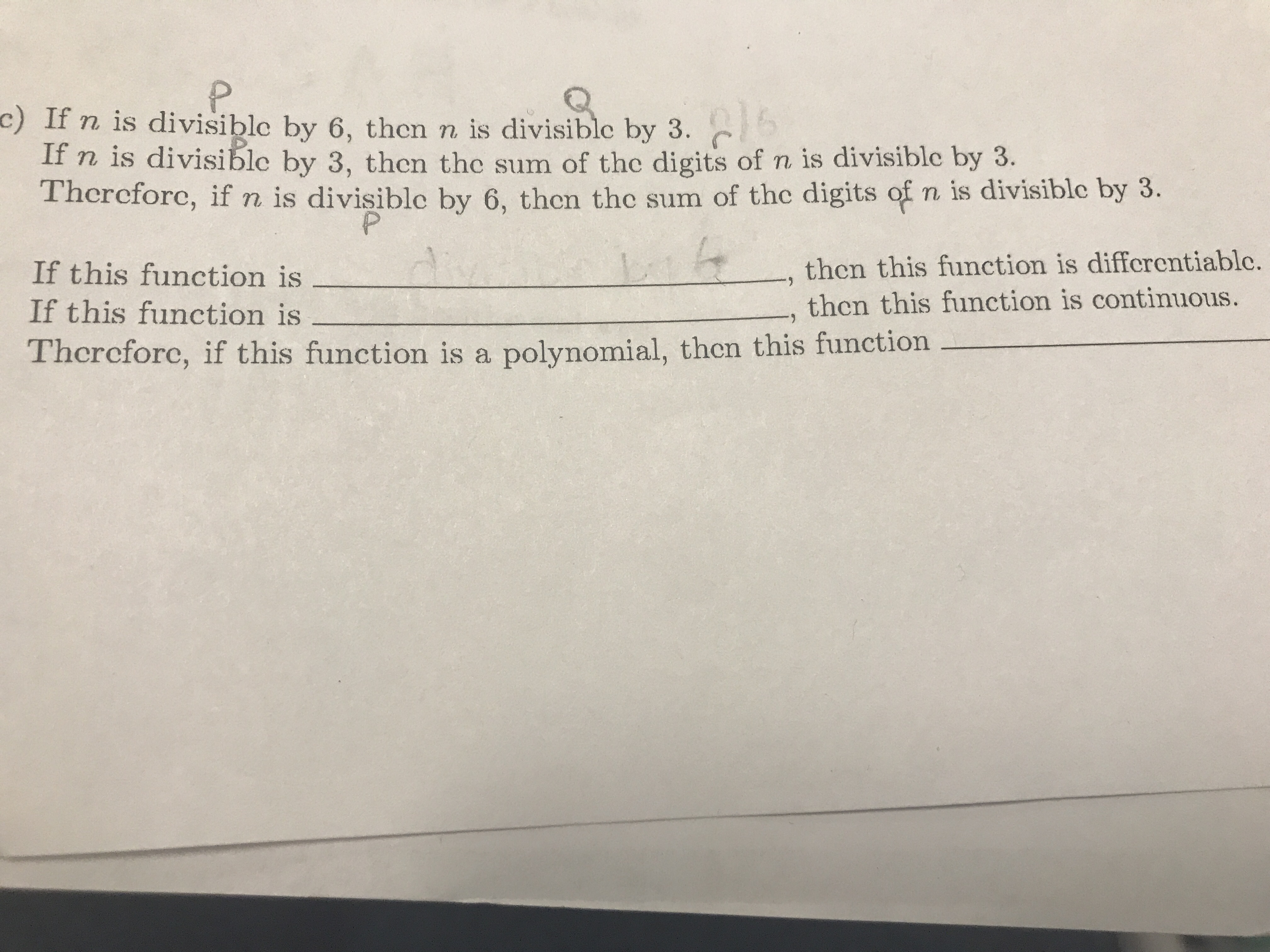# c) If n is divisible by 6, then n is divisible by 3. If n is divisible by 3, then the sum of the digits of n is divisible by 3. Thercforc, if n is divisible by 6, then the sum of the digits of n is divisible by 3. If this function is If this function is Therefore, if this function is a polynomial, then this function , then this finction is differentiable. then this function is continuous.

Questionhelp_outlineImage Transcriptionclosec) If n is divisible by 6, then n is divisible by 3. If n is divisible by 3, then the sum of the digits of n is divisible by 3. Thercforc, if n is divisible by 6, then the sum of the digits of n is divisible by 3. If this function is If this function is Therefore, if this function is a polynomial, then this function , then this finction is differentiable. then this function is continuous. fullscreen

### Want to see the step-by-step answer?